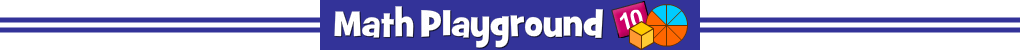AdvertisementModel and solve algebra equations.
Model Algebra Equations - Learning Connections
Essential Skills
Mental Math - solving visual equations

Common Core Connection for Grades 5+
Write, read, and evaluate expressions in which letters stand for numbers.
Understand that a variable can represent an unknown number
Understand solving an equation or inequality as a process of answering a question: which values from a specified set, if any, make the equation or inequality true?
More Math Games to Play